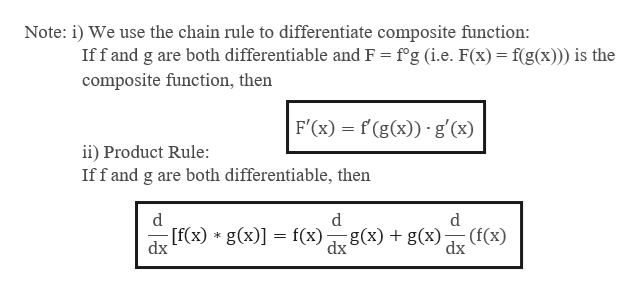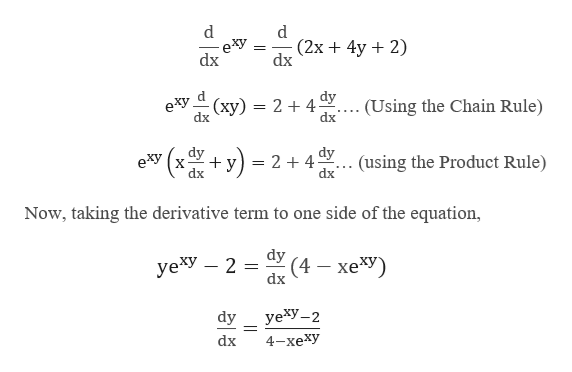# Let exy = 2x + 4y + 2.Use implicit differentiation to find the derivative of y with respect to x. dydx = xA yB exy − CD − xF yG exy , whereA = B = C = D = F = G =

Question
57 views

Let exy = 2x + 4y + 2.

Use implicit differentiation to find the derivative of y with respect to x.

 dy dx

=

 xA yB exy − C D − xF yG exy

, where

A =
B =
C =
D =
F =
G =

check_circle

star
star
star
star
star
1 Rating
Step 1

Given:

Step 2

In implicit differentiation, we differentiate each side of an equation by treating one of the variables as a function of the other. We treat y as an implicit function of x.help_outlineImage TranscriptioncloseNote: i) We use the chain rule to differentiate composite function: Iff and g are both differentiable and F = f'g (i.e. F(x) f(g(x)) is the composite function, then F'(x) f(g(x)) g'(x) ii) Product Rule: Iff and g are both differentiable, then d d d [f(x) g(x)f(x)g(x) + g(x) dx (f(x) dx = dx fullscreen
Step 3

Now differentiate both side...help_outlineImage Transcriptionclosed d (2x4y 2) dx dx dy eху (ху) — 2+4 dx (Using the Chain Rule) dy х dx +y) dy (using the Product Rule) = 2 + 4- e*y dx Now, taking the derivative term to one side of the equation, dy (4 xe*y) yе*У — 2 dx уеху—2 dy dx 4-хеху fullscreen

### Want to see the full answer?

See Solution

#### Want to see this answer and more?

Solutions are written by subject experts who are available 24/7. Questions are typically answered within 1 hour.*

See Solution
*Response times may vary by subject and question.
Tagged in

### Derivative